# RBSE Solutions For Class 12 Physics Chapter 6: Electric Circuit | Textbook Important Questions & Answers

The answers are provided for all the questions of Chapter 6 Physics of RBSE Class 12. Students can go through these questions to understand the concepts better and score well in the board examination and entrance examinations for various professional courses. These solutions are provided by the team of experts and offer the best and accurate solutions to the questions. Do check BYJU’S RBSE Class 12 solutions page to get the answers for textbook questions of all subjects.

## Multiple Choice Questions

Q1: Kirchhoff’s first and second laws are based on:

1. Charge and Energy Conservation Laws
2. Current and Energy Conservation Laws
3. Mass and Charge Conservation Laws
4. None of these

Q2: In a Wheatstone bridge, the position of battery and galvanometer is interchanged, then the new balanced position will be:

1. Unchanged
2. Changed
3. Can’t say anything
4. May or may not change; it depends upon the galvanometer and battery

Q3: Potentiometer is a type of instrument by which potential difference can be measured, then its resistance will be

1. Zero
2. Infinite
3. Uncertain
4. Depends on external resistance

Q4: The physical quantity which cannot be measured with the help of potentiometer is

1. EMF of cell
2. Inductance and capacitance
3. Resistance
4. Electric current

Q5: Temperature coefficient of resistance of potentiometer wire should be

1. High
2. Low
3. Negligible
4. Infinite

Q1: Write the mathematical form of Kirchhoff’s junction law.

Answer: According to Kirchhoff’s junction law sum of the currents in or out of a junction =0

Mathematically it can be expressed asI=0

Q2: On what conservation law Kirchhoff’s voltage rule is based?

Answer: Kirchhoff’s voltage rule is based on the Law of conservation of energy.

Q3: Write the condition of the balanced state of the Wheatstone bridge.

Answer: When the Wheatstone bridge is balanced then the ratio of the resistance of ratio arm is equal to =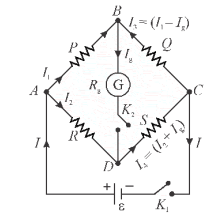Q4: What is the principle of the meter bridge?

Meter bridge works on the principle of Wheatstone bridge.

Q5: How does the potential gradient of the wire of potentiometer depend on the temperature?

When the temperature of the wire has increased the resistance of the wire also increases so the potential gradient also gets affected.

Q6: If the emf of the unknown cell in the secondary circuit is greater than the emf of the primary circuit of potentiometer then what will happen?

The null point cannot be found on the potentiometer wire.

Q7: Write down the definition of the potential gradient.

Answer: The potential drop per unit length of the wire is called the potential gradient of the potentiometer.

Q8: Why should the cross-sectional area of the wire be uniform in a potentiometer?

Answer: The cross-sectional area of the wire should be uniform so that the potential gradient remains constant at all the positions.

Q9: Which cell can be used for standardisation of the potentiometer other than Daniel cell?

Q10: How can the sensitivity of the potentiometer be increased?

Answer: The sensitivity of the wire can be increased by increasing the length of the wire of the potentiometer.

Q11: Length of the potentiometer wire is 10m. A standard cell of 1.1 V is balanced at 8.8m length. How much maximum potential difference can be measured with the help of this potentiometer?

Maximum potential difference

V=kl=0.125 x 10 = 12.5volt

Q12: Why copper wire can not be used in a potentiometer?

Answer: The temperature coefficient of resistance is large and specific resistance is less for copper wire. Therefore copper wire cannot be used in the potentiometer.

Q13: The potential gradient of the wire of potentiometer is 0.3 V/m. In the calibration of ammeter, the potential difference across 1Ω coil is balanced at 1.5 lengths. If the reading of ammeter is 0.28A then find out the error in the reading of ammeter.

Virtual current measure by ammeter I=0.28 A

k=0.3/1

k=0.3

Potential difference V=kl

V= 0.3*1.5= 0.45 volt

The current I1=V/R

I1= 0.45/1=0.45 Amp

Thus the error in the reading of Ammeter

ΔI = I-I1

= 0.28 – 0.45=-0.17 Amp

Q1: State Kirchhoff’s junction Law and Loop Law

Kirchhoff’s Junction Law

In an electric circuit, the algebraic sum of current at any junction point must be zero”

Mathematically it can be expressed as

I=0

The sum of the currents entering a junction is equal to the sum of the currents leaving out at the junction. The law is called Kirchhoff’s law or Junction Law

Kirchhoff’s Loop Law

According to this Law, the algebraic sum of the potential in a closed-loop must be zero.

v=0

The algebraic sum of the emf’s in any loop of the circuit is equal to the sum of the voltages across the resistance.

Q2: How is the resistance of a wire determined with the help of a meter bridge? Obtain the required formula and draw a circuit diagram.

Metre Bridge is an application of Wheatstone Bridge.

Metre bridge apparatus is also known as a slide wire bridge. It is fixed on the wooden block and consists of a long wire with a uniform cross-sectional area. It has two gaps formed using thick metal strips to make the Wheatstone bridge.

Necessary connections are done and once we are sure about the connections then slide the jockey over the meter bridge wire and find the null point. If the null deflection is shown at point D. This is the balanced condition of the Wheatstone bridge. Then the part AD acts as the resistance X.

Then according to Wheatstone’s principle, we have:

X/R = l/(100 – l)

The unknown resistance can be calculated as: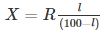Where l is the distance from A to D and R is the known resistance.

Knowing the values of l and R we can calculate unknown resistance.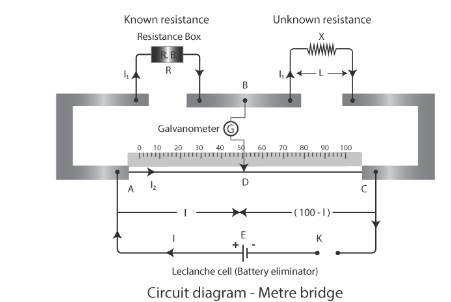Q3: What is Wheatstone bridge, derive its balanced condition using Kirchoff’s law.

A Wheatstone bridge circuit consists of four arms of which two arms consist of known resistances while the other two arms consist of an unknown resistance and a variable resistance. The circuit also consists of a galvanometer and an electromotive force source. The emf source is attached between points a and b while the galvanometer is connected between the points c and d. The current that flows through the galvanometer depends on the potential difference across it.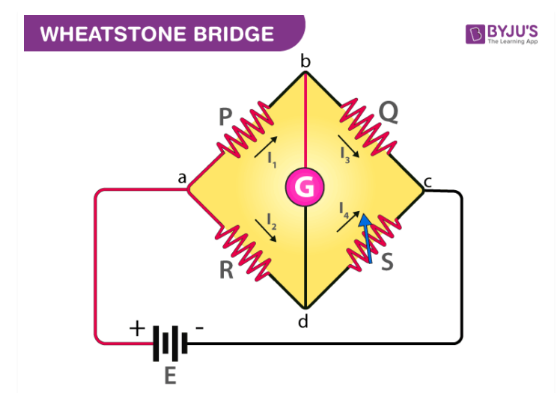Wheatstone’s Bridge derivation

The condition for a balanced Wheatstone bridge can be derived from Kirchoff’s law. Let the current in the galvanometer be Ig and its resistance is Rg.

Applying Kirchoff’s loop rule in the loop abda,

I1P + IgRg– I2R = 0————————-(1)

Applying Kirchoff’s loop rule in the loop bcdb,

(I1 – Ig)Q – (I2+ Ig)S – IgRg= 0 ———–(2)

In the balancing condition of the bridge Ig=0. Hence, the equation 1 and 2 becomes

I1P = I2R

I1Q = I2S

Dividing the above equation we get

P/Q = R/S

This is the condition for a balanced Wheatstone bridge.

Q4: What is a potential gradient? On what factors does it depend?

Answer: The fall of the potential per unit length is called the potential gradient, it is represented as x

x = є/L

Where є is the emf of the battery and L is the length of the potentiometer wire is L.The SI unit of the potential gradient is volt/m

Factors on which potential gradient depends are as follows

1. The potential gradient is directly proportional to the current I and specific resistance ρ and inversely proportional to the area of cross-section A of the wire.
2. The potential gradient depends on the emf of the battery connected in the primary circuit, length of the wire and internal resistance of the battery.

Q5: What do you mean by the standardisation of potentiometer? Explain it by drawing a circuit diagram.

The steps to find the exact potential gradient of the potentiometer is called standardisation of potentiometer.

For this procedure, a standard cell of known emf is connected to the secondary circuit. The emf of the standard cell remains constant for a long time. Daniel cell or cadmium cell is generally used as the standard cell. The emf of standard cell ranges from 1.0186 V and 1.08 V at 20-degree celsius.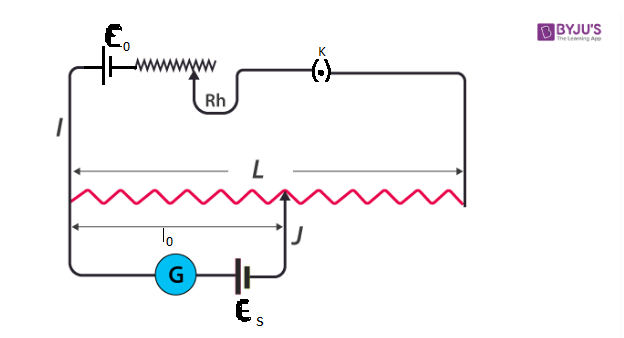For finding the potential gradient the jockey is slid on the wire till the galvanometer reading shows zero. If the emf of the standard cell is єs then according to the principle of galvanometer

Єs= xl0

x=Єs/l0

Where l0 is the balancing length.

Q6: What do you mean by the sensitivity of a potentiometer? How can we increase it?

The sensitivity of potentiometer is defined as the smallest potential difference that is measured by using a potentiometer.

The sensitivity of potentiometer can be increased by:

• Increasing the length of the potentiometer wire
• By reducing the current in the circuit by using a rheostat

Q7: Why do we try to obtain the null point near the middle of metre bridge wire?

Answer: The metre bridge is most sensitive when the four resistance forming the Wheatstone bridge are equal. This is possible only when the null point is near the middle of the meter bridge.

Q8: Why shouldn’t the current through the potentiometer wire be passed for a long time?

Answer: When the current is passed for a long time then the wire will be heated up and will change the resistance of the wire. The potential per unit length of the wire will also change.

Q9: Why is the current in the primary circuit of the potentiometer kept constant?

Answer: If the value of the current is changed in the primary circuit, the potential gradient will change and this causes error in measurement.

Q10: Write two precautions while using a potentiometer.

1. Current should not be passed through the potentiometer wire for a long time. When the current is passed for a long time then the wire will be heated up and will change the resistance of the wire. The potential per unit length of the wire will also change.

2. Area of the cross-section of the wire should be constant otherwise potential radiant will vary

Q11: What do you mean by calibration of a voltmeter? Draw the necessary circuit diagram.

The method to check the correctness of the measured value of voltmeter is carried out using a potentiometer and it is called calibration of a voltmeter.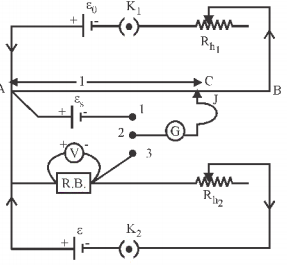Q12: Draw the required circuit diagram for the measurement of low resistance with the help of a potentiometer.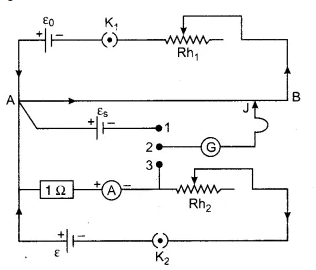## Essay Type Questions

Q1: State Kirchoff’s loop rule and junction rule. With the help of these rules deduce the condition of a balanced Wheatstone bridge. Draw the necessary diagram.

Kirchhoff’s junction Law or Kirchhoff’s Current Law

The total current entering a junction or a node is equal to the charge leaving the node as no charge is lost.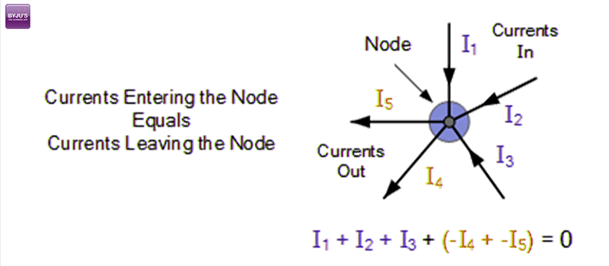Kirchhoff’s Loop Law or Kirchhoff’s Voltage Law

The voltage around a loop equals the sum of every voltage drop in the same loop for any closed network and also equals zero.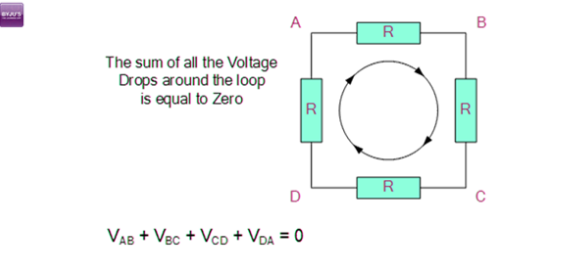Condition For Wheatstone Bridge: A Wheatstone bridge circuit consists of four arms of which two arms consists of known resistances while the other two arms consist of an unknown resistance and a variable resistance. The circuit also consists of a galvanometer and an electromotive force source. The emf source is attached between points a and b while the galvanometer is connected between the points c and d. The current that flows through the galvanometer depends on the potential difference across it.Wheatstone’s Bridge derivation

The condition for a balanced Wheatstone bridge can be derived from Kirchoff’s law. Let the current in the galvanometer be Ig and its resistance is Rg.

Applying Kirchoff’s loop rule in the loop abda,

Applying Kirchoff’s loop rule in the loop abda,

I1P + IgRg– I2R = 0————————-(1)

Applying Kirchoff’s loop rule in the loop bcdb,

(I1 – Ig)Q – (I2+ Ig)S – IgRg= 0 ———–(2)

In the balancing condition of the bridge Ig=0. Hence, the equation 1 and 2 becomes

I1P = I2R

I1Q = I2S

Dividing the above equation we get

P/Q = R/S

This is the condition for a balanced Wheatstone bridge.

Q2: What is a potentiometer? Explain its principle. Draw the necessary circuit diagram.

Potentiometer

The potentiometer is a device used to measure the emf of the cell or potential difference between two points in the circuit.

Principle of potentiometer

The principle of the potentiometer is that the potential dropped across a segment of a wire of uniform cross-section carrying a constant current is directly proportional to its length. The unknown potential difference is found by comparing it with the known potential difference of the wire. When there is no deflection in the galvanometer the unknown potential difference will be equal to the known potential difference. This is the principle of the potentiometer

To understand the principle an electric circuit is made as shown in the figure.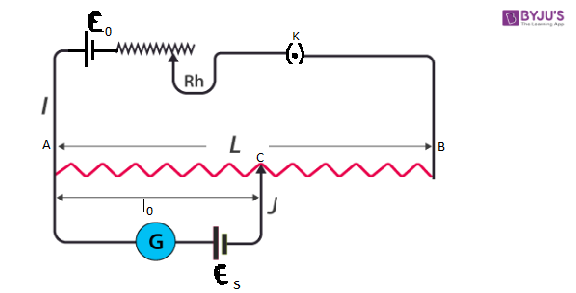A battery of emf Є0, rheostat and key connected to the potentiometer wire AB. This is the primary circuit.

In the secondary circuit, the positive terminal of wire is connected to A and the negative terminal is connected to the jockey.

The potential gradient x is given by the

x=Є0/L————————(1)

Є0 = xL =V

Where L is the length of the potentiometer wire.

If the resistance of the potentiometer wire is R and the current flowing in the potentiometer wire is I, then

V=IR

By substituting V in equation 1 we get

x = I(R/L)

x = IRm

Where Rm =R/L is the resistance per unit length.

Now we consider a point on the potentiometer wire at a distance l0 from A. Then the potential difference between A and B is given by V=xl0

Since we know the value of x and l0 the potential difference V can be calculated. If galvanometer shows zero deflection at a distance l0 from A, then the distance l0 is the balancing distance on potentiometer wire of emf Єs. In this situation, unknown emf Єs is equal to the known potential difference V.